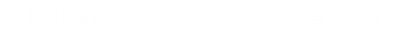# IMS Risk Calculations

IMS Risk Score calculations. XML Functions

## Goal

The Risk Rating takes two scores (Impact Severity and Frequency) and then references a matrix to produce the final score in this example.The first score has seven possibilities (A-H), and then takes the most serve rating (in this case H is the most severe) and then references the Frequency 1-4 and that produces the Risk Rating score.

Below is the first score to calculate. The first output will be a letter A - H.The below is an example of the structure of the code required. It uses a switch statement to test what was selected and outputs the corresponding letter score.

function fxEconomicSev(){

switch(s("EconomicSev")){
case "1. <\$250K":
value="A";
break;
case "2. \$250K to <\$1MM":
value="B";
break;
case "3. \$1MM to <\$5MM":
value="C";
break;
case "4. \$5MM to <\$10MM":
value="D";
break;
case "5. \$10MM to <\$50MM":
value="E";
break;
case "6. \$50MM to <\$350MM":
value="F";
break;
case "7. \$350MM to <\$1B":
value="G";
break;
case "8. > \$1B":
value="H";
break;
default:
value = "";
}
return value;
}

Then you would do that for all the remaining impact/severities as in the attached XML file.

Then another function is required to see what the impact was. It takes the letter H for example if it's the most severe impact.

Remember to declare any new variable or working variables to product the returned value. A switch statement is an exception and generates a variable without declaration.

The library lo dash is used here with the _.includes method. It searches the array for the highest letter in order with the switch statement.

https://lodash.com/docs/#includes

function fxImpactlevel(){

var value1="";
var valtemp= new Array();
var value="";
if(C7.defined(s("EconomicSev")) && s("ImpactEconomic")=== true)
valtemp.push(fxEconomicSev());
if(C7.defined(s("RegulatorySev")) && s("ImpactRegulatory")=== true)
valtemp.push(fxRegulatorySev());
if(C7.defined(s("ReputationalSev")) && s("ImpactReputational")=== true)
valtemp.push(fxReputationalSev());
if(C7.defined(s("PLSev")) && s("ImpactPL")=== true)
valtemp.push(fxPLSev());
if(C7.defined(s("BSReclassSev")) && s("ImpactBSReclass")=== true)
valtemp.push(fxBSReclassSev());
if(C7.defined(s("RWASev")) && s("ImpactRWA")=== true)
valtemp.push(fxRWASev());
if(C7.defined(s("RegCapSev")) && s("ImpactRegCap")=== true)
valtemp.push(fxRegCapSev());

switch(true){
case C7.equals(_.includes(valtemp,"H"),C7.true):
value="H";
break;
case C7.equals(_.includes(valtemp,"G"),C7.true):
value="G";
break;
case C7.equals(_.includes(valtemp,"F"),C7.true):
value="F";
break;
case C7.equals(_.includes(valtemp,"E"),C7.true):
value="E";
break;
case C7.equals(_.includes(valtemp,"D"),C7.true):
value="D";
break;
case C7.equals(_.includes(valtemp,"C"),C7.true):
value="C";
break;
case C7.equals(_.includes(valtemp,"B"),C7.true):
value="B";
break;
case C7.equals(_.includes(valtemp,"A"),C7.true):
value="A";
break;
default:
value = "";
}

return value;

}

Then the Risk Rating is ready to be looked up. Now that the letter is know and the frequency is known.

function fxRiskRating(){
var value="";

if(C7.equals(fxImpactlevel(),"A") && C7.equals(s("RLSFrequency"),"1. Very Frequent (within the next 6 months or quarterly on average)"))
value=1;

if(C7.equals(fxImpactlevel(),"A") && C7.equals(s("RLSFrequency"),"2. Frequent (within the next 6-24 months or annually on average)"))
value=2;

if(C7.equals(fxImpactlevel(),"B") && C7.equals(s("RLSFrequency"),"1. Very Frequent (within the next 6 months or quarterly on average)"))
value=2;
if(C7.equals(fxImpactlevel(),"A") && C7.equals(s("RLSFrequency"),"3. Occasional (within the next 2-5 years)"))
value=3;
if(C7.equals(fxImpactlevel(),"B") && C7.equals(s("RLSFrequency"),"2. Frequent (within the next 6-24 months or annually on average)"))
value=3;
if(C7.equals(fxImpactlevel(),"C") && C7.equals(s("RLSFrequency"),"1. Very Frequent (within the next 6 months or quarterly on average)"))
value=3;
if(C7.equals(fxImpactlevel(),"A") && C7.equals(s("RLSFrequency"),"4. Infrequent (once in ~5-10 years)"))
value=4;
if(C7.equals(fxImpactlevel(),"B") || C7.equals(fxImpactlevel(),"C")  && C7.equals(s("RLSFrequency"),"3. Occasional (within the next 2-5 years)"))
value=4;
if(C7.equals(fxImpactlevel(),"C") && C7.equals(s("RLSFrequency"),"2. Frequent (within the next 6-24 months or annually on average)"))
value=4;
if(C7.equals(fxImpactlevel(),"D") && C7.equals(s("RLSFrequency"),"1. Very Frequent (within the next 6 months or quarterly on average)"))
value=4;
if(C7.equals(fxImpactlevel(),"B") || C7.equals(fxImpactlevel(),"C") && C7.equals(s("RLSFrequency"),"4. Infrequent (once in ~5-10 years)"))
value=6;
if(C7.equals(fxImpactlevel(),"D") && C7.equals(s("RLSFrequency"),"3. Occasional (within the next 2-5 years)"))
value=6;
if(C7.equals(fxImpactlevel(),"D") || C7.equals(fxImpactlevel(),"E") && C7.equals(s("RLSFrequency"),"2. Frequent (within the next 6-24 months or annually on average)"))
value=6;
if(C7.equals(fxImpactlevel(),"E") && C7.equals(s("RLSFrequency"),"1. Very Frequent (within the next 6 months or quarterly on average)"))
value=6;
if(C7.equals(fxImpactlevel(),"D") && C7.equals(s("RLSFrequency"),"4. Infrequent (once in ~5-10 years)"))
value=7;
if(C7.equals(fxImpactlevel(),"E") && C7.equals(s("RLSFrequency"),"3. Occasional (within the next 2-5 years)"))
value=7;
if(C7.equals(fxImpactlevel(),"F") && C7.equals(s("RLSFrequency"),"2. Frequent (within the next 6-24 months or annually on average)"))
value=7;
if(C7.equals(fxImpactlevel(),"F") || C7.equals(fxImpactlevel(),"G") && C7.equals(s("RLSFrequency"),"1. Very Frequent (within the next 6 months or quarterly on average)"))
value=7;
if(C7.equals(fxImpactlevel(),"E") || C7.equals(fxImpactlevel(),"F") || C7.equals(fxImpactlevel(),"G") || C7.equals(fxImpactlevel(),"H") && C7.equals(s("RLSFrequency"),"4. Infrequent (once in ~5-10 years)"))
value=8;
if(C7.equals(fxImpactlevel(),"F")|| C7.equals(fxImpactlevel(),"G") || C7.equals(fxImpactlevel(),"H") && C7.equals(s("RLSFrequency"),"3. Occasional (within the next 2-5 years)"))
value=8;
if(C7.equals(fxImpactlevel(),"G") || C7.equals(fxImpactlevel(),"H") && C7.equals(s("RLSFrequency"),"2. Frequent (within the next 6-24 months or annually on average)"))
value=8;
if(C7.equals(fxImpactlevel(),"H") && C7.equals(s("RLSFrequency"),"1. Very Frequent (within the next 6 months or quarterly on average)"))
value=8;

return value;
}

The if statements filter out by the lowest to highest rating. So the highest rating is caught at the end of the list of if statements.

This is what was achieved and what was omitted in this how-to.